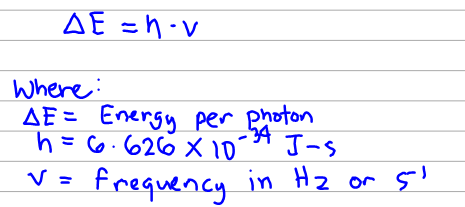🤓 Based on our data, we think this question is relevant for Professor Lackey's class at UA.

# Solution: Calculate the energy of a photon of electromagnetic radiation at each of the following frequencies (in J).i) 103.7 MHz (typical frequency for FM radio broadcasting)ii) 1100 kHz (typical frequency for AM radio broadcasting) (assume four significant figures)

###### Problem

Calculate the energy of a photon of electromagnetic radiation at each of the following frequencies (in J).View Complete Written Solution